## Autonomous Driving

Problem Statement : Can you predict vehicle angle in different settings?

The training set consists of over 4000 images of the street taken by a camera attached to the top of a car. These images have various other cars in them (there can be none or many). We have information on the following `pose` information of each of these cars in the images:

`model_type, yaw, pitch, roll, x, y, z`

We are also provided with the camera intrinsics to convert camera coordinates to image coordinates. Some cars might be too far off so we've been provided with masks to get rid of insignificant cars (both in test and train data). Additionally, we're provided with 3D models of each car type (which we may not need to use!).

Our target is to predict the following pose information for each of the test images (Note we don't need to predict model_type):

`yaw, pitch, roll, x, y, z, confidence in prediction`

#### Library imports

In :
``````import numpy as np
import pandas as pd
import cv2
from tqdm import tqdm
import matplotlib.pyplot as plt
import seaborn as sns
from functools import reduce
import os
from scipy.optimize import minimize
import plotly.express as px
import matplotlib.image as mpimg

from sklearn.model_selection import train_test_split
from sklearn.linear_model import LinearRegression
from sklearn.metrics import mean_absolute_error, mean_squared_error

import torch
import torch.nn as nn
import torch.nn.functional as F
import torch.optim as optim
from torch.optim import lr_scheduler
from torch.utils.data import Dataset, DataLoader
from torchvision import models
from torchvision import transforms, utils

PATH = '../../auto_driving/'
os.listdir(PATH)
``````
Out:
``````['test_images',
'.DS_Store',
'car_models_json',
'camera',
'car_models',
'train.csv',
'train_images',
'test_masks',
'pku-autonomous-driving.zip',
'train_masks',
'jupyter',
'sample_submission.csv']``````

#### Load Data

`train.csv` consists of the pose information for each image for each car present in that image

We also load the camera intrinsic parameters to be able to use it later for coord conversions

In :
``````train = pd.read_csv(PATH + 'train.csv')
test = pd.read_csv(PATH + 'sample_submission.csv')

# From camera.zip
camera_matrix = np.array([[2304.5479, 0,  1686.2379],
[0, 2305.8757, 1354.9849],
[0, 0, 1]], dtype=np.float32)
train.head()
``````
Out:
In :
``train.shape``
Out:
``(4262, 2)``

#### Read Images

In :
``````def img_read(path):
return mpimg.imread(path)``````
In :
``````plt.figure(figsize=(15,8))
img = img_read(PATH + 'train_images/ID_337ddc495' + '.jpg')
imgplot = plt.imshow(img)
img_shape = img.shape
print("Shape of image: ", img_shape)``````
```Shape of image: (2710, 3384, 3) ```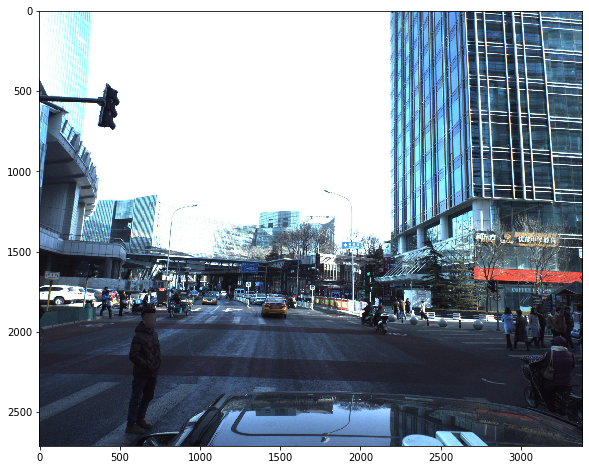#### Extract pose info of each car

In our `train.csv` we have a single string representing pose info for all cars in the image. For example, an image having two cars has the following entry:

`5 0.5 0.5 0.5 0.0 0.0 0.0 32 0.25 0.25 0.25 0.5 0.4 0.7`

We extract out the details for each car in a particular image to get a list of dictionaries

In :
``````def str_to_coords(s, keys=['model_type', 'yaw', 'pitch', 'roll', 'x', 'y', 'z']):
'''
Input:
s: PredictionString (e.g. from train dataframe)
keys: array of things to extract from the string
Output:
list of dicts with given keys
'''
coords = []
for car in np.array(s.split()).reshape([-1,7]):
coord = dict(zip(keys, car.astype('float')))
if 'model_type' in coord:  # model_type needs to be integer
coord['model_type'] = int(coord['model_type'])
coords.append(coord)
return coords``````
In :
``````inp = train['PredictionString']
print('Example input:\n', inp)
print()
print('Output:\n', str_to_coords(inp))``````
```Example input: 16 0.254839 -2.57534 -3.10256 7.96539 3.20066 11.0225 56 0.181647 -1.46947 -3.12159 9.60332 4.66632 19.339 70 0.163072 -1.56865 -3.11754 10.39 11.2219 59.7825 70 0.141942 -3.1395 3.11969 -9.59236 5.13662 24.7337 46 0.163068 -2.08578 -3.11754 9.83335 13.2689 72.9323 Output: [{'model_type': 16, 'yaw': 0.254839, 'pitch': -2.57534, 'roll': -3.10256, 'x': 7.96539, 'y': 3.20066, 'z': 11.0225}, {'model_type': 56, 'yaw': 0.181647, 'pitch': -1.46947, 'roll': -3.12159, 'x': 9.60332, 'y': 4.66632, 'z': 19.339}, {'model_type': 70, 'yaw': 0.163072, 'pitch': -1.56865, 'roll': -3.11754, 'x': 10.39, 'y': 11.2219, 'z': 59.7825}, {'model_type': 70, 'yaw': 0.141942, 'pitch': -3.1395, 'roll': 3.11969, 'x': -9.59236, 'y': 5.13662, 'z': 24.7337}, {'model_type': 46, 'yaw': 0.163068, 'pitch': -2.08578, 'roll': -3.11754, 'x': 9.83335, 'y': 13.2689, 'z': 72.9323}] ```

### EDA

#### Number of cars in each photo

There's an average of 11 cars in each photo

In :
``````car_nums = [len(str_to_coords(x)) for x in train['PredictionString']]
plt.figure(figsize=(15,8))
sns.countplot(car_nums)

print("Average number of cars: ", np.mean(car_nums))``````
```Average number of cars: 11.657437822618489 ```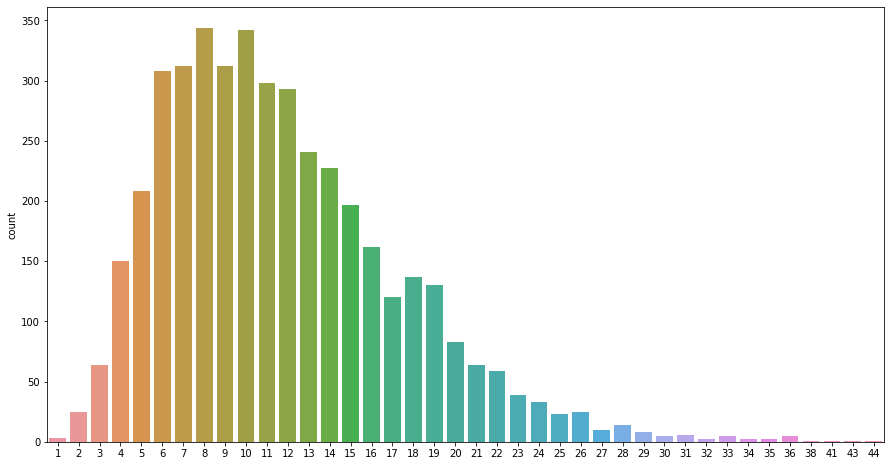#### Distribution of x, y, z, yaw, pitch, roll (with respect to camera)

In :
``````## Dataframe of all cars present across all images

cars = pd.DataFrame()
for col in ['x', 'y', 'z', 'yaw', 'pitch', 'roll']:
arr = []
for ps in train['PredictionString']:
coords = str_to_coords(ps)
arr += [c[col] for c in coords]
cars[col] = arr

print('Total number of cars:', len(cars))
cars.head()

``````
```Total number of cars: 49684 ```
Out:
##### Distribution of x
In :
``````plt.figure(figsize=(15,8))
plot = plt.hist(cars['x'], color = 'blue', edgecolor = 'black',
bins = 500)``````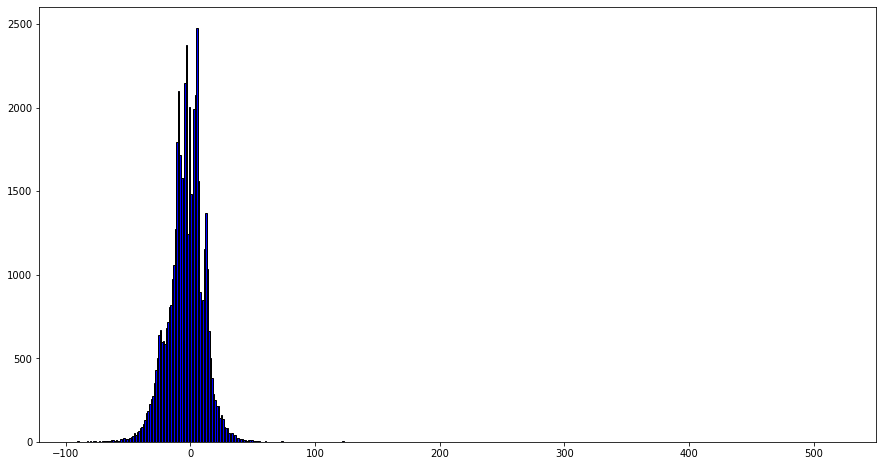##### Distribution of y
In :
``````plt.figure(figsize=(15,8))
plot = plt.hist(cars['y'], color = 'blue', edgecolor = 'black',
bins = 500)``````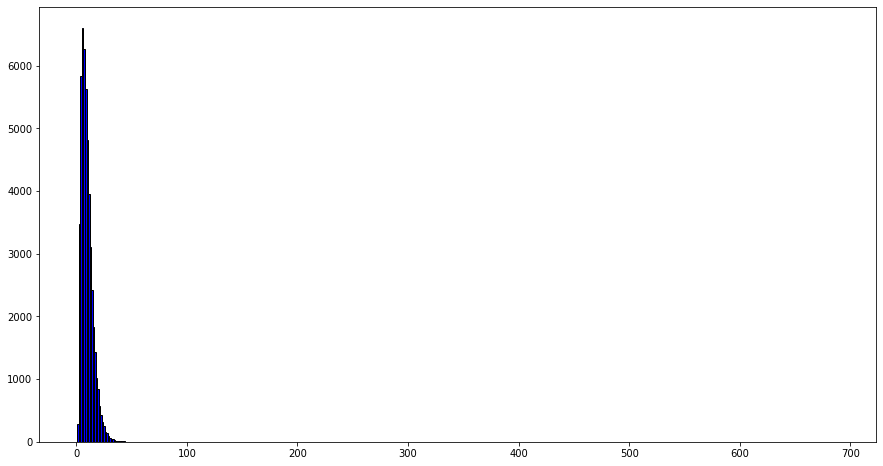##### Distribution of z
In :
``````plt.figure(figsize=(15,8))
plot = plt.hist(cars['z'], color = 'blue', edgecolor = 'black',
bins = 500)``````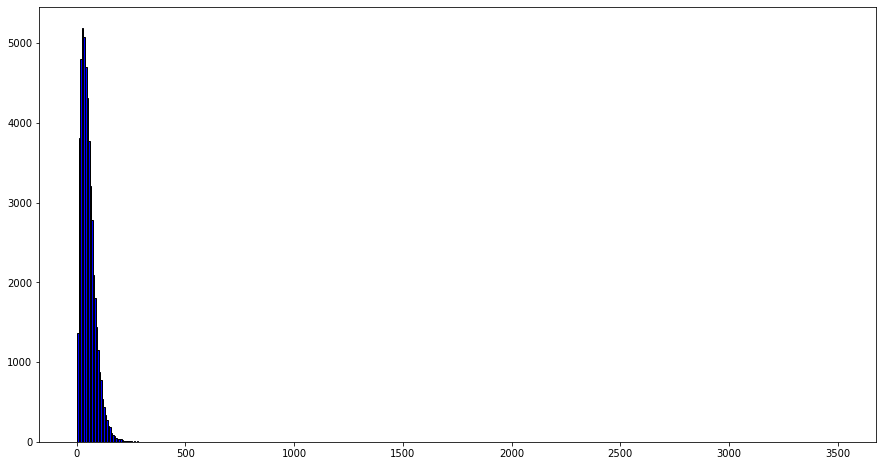##### Distribution of yaw

Yaw is the rotation along the y-axis

In :
``````plt.figure(figsize=(15,8))
plot = plt.hist(cars['yaw'], color = 'blue', edgecolor = 'black',
bins = 500)``````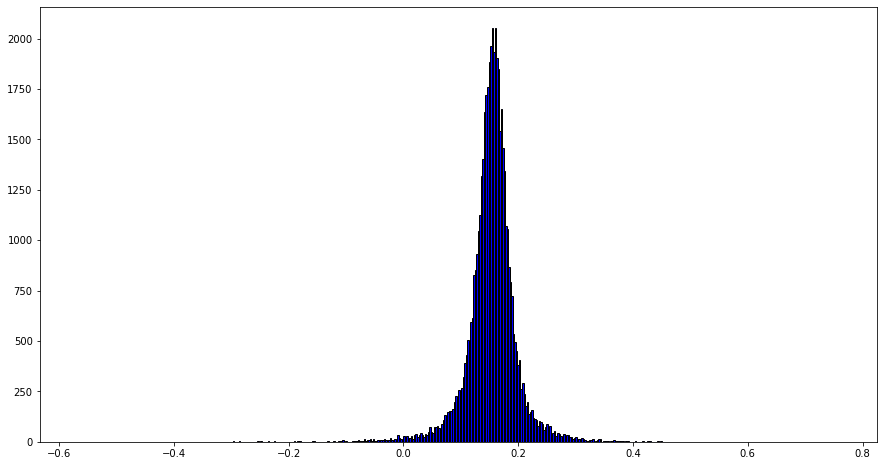##### Distribution of pitch

Pitch is the rotation along the x-axis. The distribution implies that there are upside down cars :P , the conclusion we can draw from this is that pitch and yaw are interchanged in this dataset

In :
``````plt.figure(figsize=(15,8))
plot = plt.hist(cars['pitch'], color = 'blue', edgecolor = 'black',
bins = 500)``````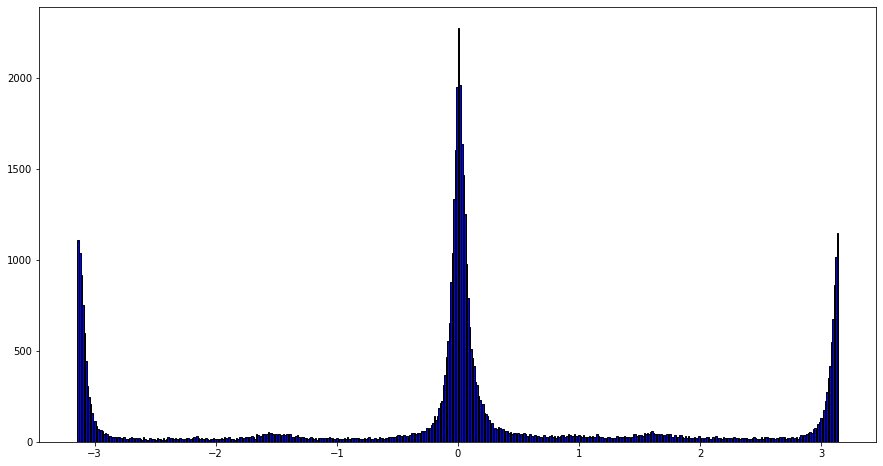##### Distribution of roll

Roll is the rotation along the z-axis. From this graph we see most values at the extremes indicating upside down cars. We can rotate by pi to correct this

In :
``````plt.figure(figsize=(15,8))
plot = plt.hist(cars['roll'], color = 'blue', edgecolor = 'black',
bins = 500)``````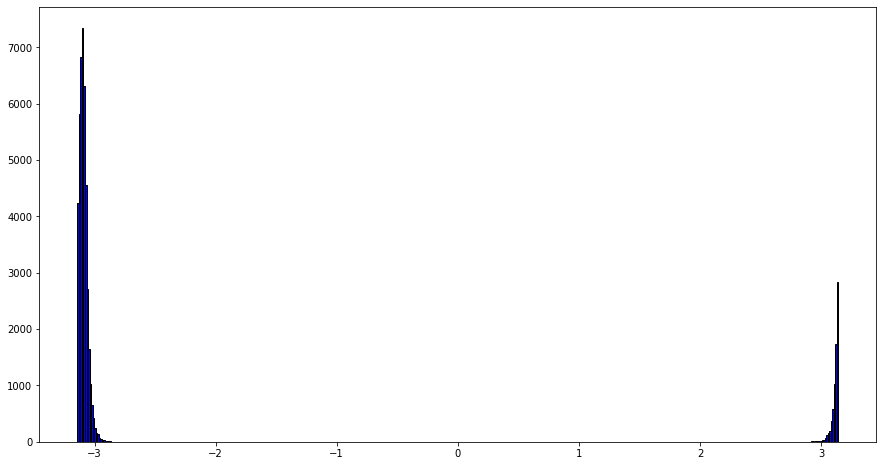In :
``````def rotate(x, angle):
x = x + angle
x = x - (x + np.pi) // (2 * np.pi) * 2 * np.pi
return x

plt.figure(figsize=(15,6))
sns.distplot(cars['roll'].map(lambda x: rotate(x, np.pi)), bins=500);
plt.xlabel('roll rotated by pi')
plt.show()
``````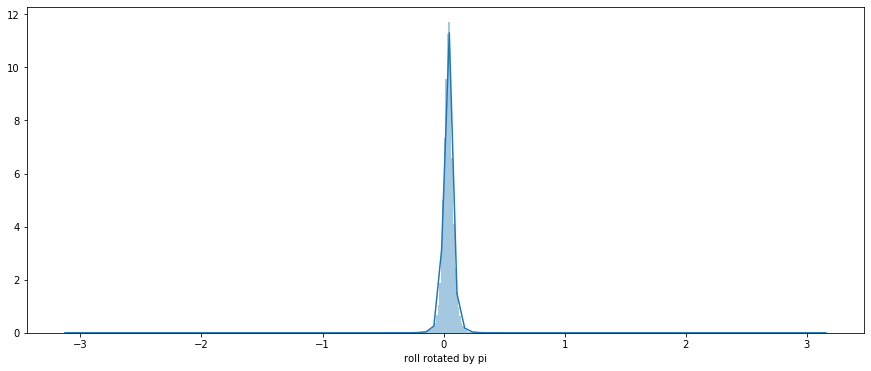### 2D visualization

Here we try to get the corrected x and y coordinates using the camera parameters and plot these points on the actual image to see if we get expected results

In [ ]:
``````def get_coords(img):

``````
In :
``import jovian``
In [ ]:
``jovian.commit()``
```[jovian] Saving notebook.. ```
In [ ]:
`` ``